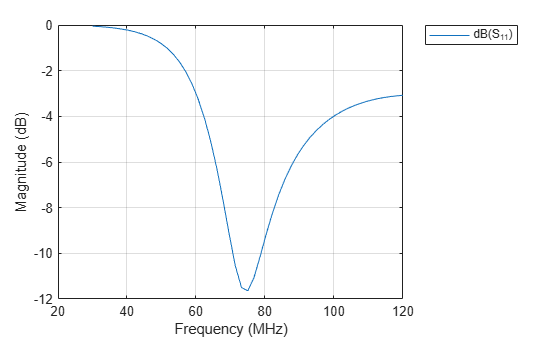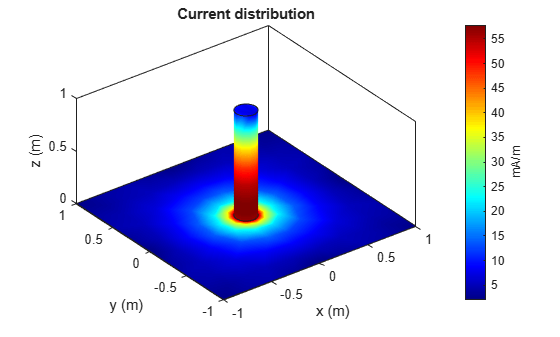# monopoleCylindrical

Create cylindrical monopole antenna over rectangular ground plane

## Description

The `monopoleCylindrical` object is a cylindrical monopole antenna mounted over a rectangular ground plane. This antenna is useful for designing thicker monopole antennas. These antennas are mostly used in wireless mobile communication due to their broadband characteristics and simple design.## Creation

### Syntax

``ant = monopoleCylindrical``
``ant = monopoleCylindrical(Name,Value)``

### Description

example

``` `ant = monopoleCylindrical` creates a cylindrical monopole antenna object with default dimensions for an operating frequency of 70 MHz.```

example

````ant = monopoleCylindrical(Name,Value)` sets Properties using one or more name-value pairs. For example, ```ant = monopoleCylindrical('Radius',0.04)```, creates a cylindrical monopole antenna with a radius of 0.04 meters.```

## Properties

expand all

Height of the monopole along the z-axis, specified as a positive scalar in meters.

Example: `'Height',3`

Data Types: `double`

Radius of the monopole, specified as a positive scalar in meters.

Example: `'Radius',0.05`

Data Types: `double`

Ground plane length along x-axis, specified as a positive scalar in meters.

Example: `'GroundPlaneLength',4`

Data Types: `double`

Ground plane width along y-axis, specified as a positive scalar in meters.

Example: `'GroundPlaneWidth',2.5`

Data Types: `double`

Signed distance from the center along the length and the width of the ground plane, specified as a two-element vector in meters.

Example: `'FeedOffset',[2 1]`

Data Types: `double`

Determine if the monopole top is closed with a cap or left open, specified as 0 or 1. Specify 1 for a closed-top monopole, and 0 for an open-top monopole.

Example: `'ClosedEnd',1`

Data Types: `double`

Type of the metal used as a conductor, specified as a metal material object. You can choose any metal from the `MetalCatalog` or specify a metal of your choice. For more information, see `metal`. For more information on metal conductor meshing, see Meshing.

Example: `m = metal('Copper'); 'Conductor',m`

Example: `m = metal('Copper'); ant.Conductor = m`

Tilt angle of the antenna, specified as a scalar or vector with each element unit in degrees. For more information, see Rotate Antennas and Arrays.

Example: `'Tilt',90`

Example: `ant.Tilt = 90`

Example: `'Tilt',[90 90]`,```'TiltAxis',[0 1 0;0 1 1]``` tilts the antenna at 90 degrees about the two axes defined by the vectors.

Data Types: `double`

Tilt axis of the antenna, specified as:

• Three-element vector of Cartesian coordinates in meters. In this case, each coordinate in the vector starts at the origin and lies along the specified points on the X-, Y-, and Z-axes.

• Two points in space, each specified as three-element vectors of Cartesian coordinates. In this case, the antenna rotates around the line joining the two points in space.

• A string input describing simple rotations around one of the principal axes, 'X', 'Y', or 'Z'.

Example: `'TiltAxis',[0 1 0]`

Example: `'TiltAxis',[0 0 0;0 1 0]`

Example: `ant.TiltAxis = 'Z'`

Data Types: `double`

Lumped elements added to the antenna feed, specified as a `lumpedElement` object handle. You can add a load anywhere on the surface of the antenna. By default, the load is at the feed. For more information, see `lumpedElement`.

Example: `'Load',lumpedelement`, where `lumpedelement` is the load added to the antenna feed.

Example: `ant.Load = lumpedElement('Impedance',75)`

## Object Functions

 `show` Display antenna or array structure; display shape as filled patch `axialRatio` Axial ratio of antenna `beamwidth` Beamwidth of antenna `charge` Charge distribution on metal or dielectric antenna or array surface `current` Current distribution on metal or dielectric antenna or array surface `cylinder2strip` Cylinder equivalent width approximation `design` Design prototype antenna or arrays for resonance around specified frequency `efficiency` Radiation efficiency of antenna `EHfields` Electric and magnetic fields of antennas; Embedded electric and magnetic fields of antenna element in arrays `impedance` Input impedance of antenna; scan impedance of array `mesh` Mesh properties of metal or dielectric antenna or array structure `meshconfig` Change mesh mode of antenna structure `optimize` Optimize antenna or array using SADEA optimizer `pattern` Radiation pattern and phase of antenna or array; Embedded pattern of antenna element in array `patternAzimuth` Azimuth pattern of antenna or array `patternElevation` Elevation pattern of antenna or array `rcs` Calculate and plot radar cross section (RCS) of platform, antenna, or array `returnLoss` Return loss of antenna; scan return loss of array `sparameters` Calculate S-parameter for antenna and antenna array objects `strip2cylinder` Calculates equivalent radius approximation for strip `vswr` Voltage standing wave ratio of antenna

## Examples

collapse all

Create a cylindrical monopole antenna with default properties.

`ant = monopoleCylindrical`
```ant = monopoleCylindrical with properties: Height: 1 Radius: 0.0400 GroundPlaneLength: 2 GroundPlaneWidth: 2 FeedOffset: [0 0] ClosedEnd: 0 Conductor: [1x1 metal] Tilt: 0 TiltAxis: [1 0 0] Load: [1x1 lumpedElement] ```

View the antenna using the `show` function.

`show(ant)`Plot the radiation pattern of cylindrical monopole antenna at a frequency of 70 MHz.

`pattern(ant,70e6)`Create a cylindrical monopole antenna with length and radius as 1 m and 50 mm respectively.

```ant = monopoleCylindrical; ant.Height = 1; ant.Radius = 50e-3```
```ant = monopoleCylindrical with properties: Height: 1 Radius: 0.0500 GroundPlaneLength: 2 GroundPlaneWidth: 2 FeedOffset: [0 0] ClosedEnd: 0 Conductor: [1x1 metal] Tilt: 0 TiltAxis: [1 0 0] Load: [1x1 lumpedElement] ```

Visualize the antenna using `show `function.

`show(ant)`Plot the S-parameters over a frequency range of 30 MHz to 120 MHz.

```s = sparameters(ant,linspace(30e6,120e6,51)); rfplot(s)```Create cylindrical monopole antennas with an open-ended top and a closed-ended top respectively.

```ant = monopoleCylindrical("Radius",0.1); ant_ClosedEnded = monopoleCylindrical("Radius",0.1,"ClosedEnd",1);```

Calculate and plot the current distribution for a cylindrical monopole antennas at 70 MHz frequency.

`I_OpenEnded = current(ant,70e6)`
```I_OpenEnded = 3×334 complex -0.0105 + 0.0033i -0.0001 + 0.0000i 0.0063 - 0.0024i -0.0008 + 0.0004i 0.0222 - 0.0056i -0.0013 + 0.0005i -0.0575 + 0.0053i -0.0037 + 0.0017i 0.0013 - 0.0005i 0.0120 - 0.0039i 0.0005 - 0.0002i 0.0111 - 0.0034i 0.0083 - 0.0029i -0.0346 + 0.0034i -0.0021 + 0.0010i -0.0108 + 0.0034i -0.0129 + 0.0033i -0.0011 + 0.0002i -0.0007 + 0.0003i -0.0061 + 0.0023i 0.0038 - 0.0010i -0.0034 + 0.0011i -0.0064 + 0.0023i -0.0059 + 0.0022i -0.0040 + 0.0019i -0.0226 + 0.0061i -0.0216 + 0.0059i -0.0084 + 0.0029i 0.0022 - 0.0012i -0.0383 + 0.0074i -0.0084 + 0.0029i -0.0034 + 0.0017i 0.0224 - 0.0061i 0.0582 - 0.0050i 0.0155 - 0.0042i -0.0137 + 0.0044i 0.0008 + 0.0003i -0.0140 + 0.0043i 0.0580 - 0.0052i -0.0367 + 0.0032i 0.0008 - 0.0004i 0.0312 - 0.0062i 0.0007 - 0.0003i 0.0123 - 0.0020i 0.0032 - 0.0014i 0.0009 - 0.0005i -0.0112 + 0.0037i -0.0037 + 0.0017i 0.0019 - 0.0007i -0.0002 - 0.0002i 0.0096 - 0.0028i 0.0053 - 0.0020i 0.0030 - 0.0013i 0.0039 - 0.0019i 0.0073 - 0.0018i 0.0084 - 0.0030i 0.0207 - 0.0015i 0.0007 - 0.0003i 0.0081 - 0.0029i 0.0054 - 0.0018i 0.0247 - 0.0062i 0.0081 - 0.0025i 0.0027 - 0.0009i 0.0505 - 0.0044i 0.0034 - 0.0017i -0.0084 + 0.0025i -0.0189 + 0.0048i -0.0240 + 0.0061i -0.0037 + 0.0017i -0.0065 + 0.0023i 0.0246 - 0.0065i 0.0085 - 0.0030i 0.0061 - 0.0023i 0.0062 - 0.0022i 0.0008 - 0.0004i -0.0061 + 0.0017i 0.0059 - 0.0017i 0.0026 - 0.0009i -0.0009 + 0.0005i 0.0003 + 0.0001i -0.0027 + 0.0009i 0.0021 - 0.0010i 0.0058 - 0.0016i 0.0181 - 0.0018i -0.0174 + 0.0047i -0.0001 + 0.0000i 0.0620 - 0.0054i 0.0007 - 0.0002i -0.0187 + 0.0014i -0.0496 + 0.0046i -0.0039 + 0.0019i -0.0227 + 0.0040i 0.0037 - 0.0017i 0.0374 - 0.0072i 0.0039 - 0.0018i 0.0022 - 0.0011i 0.0044 - 0.0016i -0.0007 + 0.0003i 0.0084 - 0.0029i -0.0619 + 0.0056i 0.0000 + 0.0000i 0.0000 + 0.0000i 0.0000 + 0.0000i 0.0000 + 0.0000i 0.0000 + 0.0000i 0.0000 + 0.0000i 0.0000 + 0.0000i 0.0000 + 0.0000i 0.0000 + 0.0000i 0.0000 + 0.0000i 0.0000 + 0.0000i 0.0000 + 0.0000i 0.0000 + 0.0000i 0.0000 + 0.0000i 0.0000 + 0.0000i 0.0000 + 0.0000i 0.0000 + 0.0000i 0.0000 + 0.0000i 0.0000 + 0.0000i 0.0000 + 0.0000i 0.0000 + 0.0000i 0.0000 + 0.0000i 0.0000 + 0.0000i 0.0000 + 0.0000i 0.0000 + 0.0000i 0.0000 + 0.0000i 0.0000 + 0.0000i 0.0000 + 0.0000i 0.0000 + 0.0000i 0.0000 + 0.0000i 0.0000 + 0.0000i 0.0000 + 0.0000i 0.0000 + 0.0000i 0.0000 + 0.0000i 0.0000 + 0.0000i 0.0000 + 0.0000i 0.0000 + 0.0000i 0.0000 + 0.0000i 0.0000 + 0.0000i 0.0000 + 0.0000i 0.0000 + 0.0000i 0.0000 + 0.0000i 0.0000 + 0.0000i 0.0000 + 0.0000i 0.0000 + 0.0000i 0.0000 + 0.0000i 0.0000 + 0.0000i 0.0000 + 0.0000i 0.0000 + 0.0000i 0.0000 + 0.0000i ```
`current(ant,70e6)``I_ClosedEnded = current(ant_ClosedEnded,70e6)`
```I_ClosedEnded = 3×342 complex -0.0095 + 0.0039i -0.0001 + 0.0000i 0.0057 - 0.0027i -0.0007 + 0.0004i 0.0201 - 0.0069i -0.0012 + 0.0006i -0.0522 + 0.0084i -0.0034 + 0.0019i 0.0012 - 0.0006i 0.0109 - 0.0045i 0.0005 - 0.0003i 0.0101 - 0.0041i 0.0076 - 0.0034i -0.0314 + 0.0053i -0.0019 + 0.0011i -0.0098 + 0.0040i -0.0117 + 0.0041i -0.0010 + 0.0003i -0.0006 + 0.0003i -0.0056 + 0.0026i 0.0035 - 0.0012i -0.0031 + 0.0013i -0.0059 + 0.0026i -0.0054 + 0.0025i -0.0036 + 0.0021i -0.0206 + 0.0074i -0.0197 + 0.0071i -0.0076 + 0.0034i 0.0020 - 0.0013i -0.0348 + 0.0095i -0.0076 + 0.0034i -0.0031 + 0.0019i 0.0204 - 0.0073i 0.0528 - 0.0082i 0.0141 - 0.0050i -0.0125 + 0.0052i 0.0007 + 0.0003i -0.0127 + 0.0050i 0.0526 - 0.0084i -0.0333 + 0.0052i 0.0007 - 0.0004i 0.0283 - 0.0079i 0.0006 - 0.0003i 0.0112 - 0.0027i 0.0029 - 0.0016i 0.0008 - 0.0005i -0.0102 + 0.0043i -0.0034 + 0.0019i 0.0018 - 0.0008i -0.0002 - 0.0002i 0.0087 - 0.0034i 0.0048 - 0.0023i 0.0028 - 0.0014i 0.0036 - 0.0021i 0.0067 - 0.0022i 0.0076 - 0.0035i 0.0188 - 0.0026i 0.0006 - 0.0003i 0.0073 - 0.0034i 0.0049 - 0.0021i 0.0225 - 0.0075i 0.0074 - 0.0029i 0.0024 - 0.0011i 0.0458 - 0.0071i 0.0031 - 0.0019i -0.0077 + 0.0030i -0.0171 + 0.0058i -0.0218 + 0.0074i -0.0034 + 0.0019i -0.0059 + 0.0027i 0.0224 - 0.0079i 0.0078 - 0.0034i 0.0056 - 0.0026i 0.0056 - 0.0025i 0.0007 - 0.0004i -0.0055 + 0.0020i 0.0054 - 0.0020i 0.0023 - 0.0010i -0.0008 + 0.0005i 0.0003 + 0.0001i -0.0024 + 0.0011i 0.0019 - 0.0011i 0.0053 - 0.0019i 0.0164 - 0.0027i -0.0158 + 0.0057i -0.0001 + 0.0000i 0.0563 - 0.0089i 0.0006 - 0.0002i -0.0170 + 0.0024i -0.0450 + 0.0074i -0.0036 + 0.0021i -0.0206 + 0.0053i 0.0033 - 0.0019i 0.0340 - 0.0092i 0.0035 - 0.0021i 0.0020 - 0.0013i 0.0040 - 0.0018i -0.0006 + 0.0003i 0.0077 - 0.0034i -0.0562 + 0.0090i 0.0000 + 0.0000i 0.0000 + 0.0000i 0.0000 + 0.0000i 0.0000 + 0.0000i 0.0000 + 0.0000i 0.0000 + 0.0000i 0.0000 + 0.0000i 0.0000 + 0.0000i 0.0000 + 0.0000i 0.0000 + 0.0000i 0.0000 + 0.0000i 0.0000 + 0.0000i 0.0000 + 0.0000i 0.0000 + 0.0000i 0.0000 + 0.0000i 0.0000 + 0.0000i 0.0000 + 0.0000i 0.0000 + 0.0000i 0.0000 + 0.0000i 0.0000 + 0.0000i 0.0000 + 0.0000i 0.0000 + 0.0000i 0.0000 + 0.0000i 0.0000 + 0.0000i 0.0000 + 0.0000i 0.0000 + 0.0000i 0.0000 + 0.0000i 0.0000 + 0.0000i 0.0000 + 0.0000i 0.0000 + 0.0000i 0.0000 + 0.0000i 0.0000 + 0.0000i 0.0000 + 0.0000i 0.0000 + 0.0000i 0.0000 + 0.0000i 0.0000 + 0.0000i 0.0000 + 0.0000i 0.0000 + 0.0000i 0.0000 + 0.0000i 0.0000 + 0.0000i 0.0000 + 0.0000i 0.0000 + 0.0000i 0.0000 + 0.0000i 0.0000 + 0.0000i 0.0000 + 0.0000i 0.0000 + 0.0000i 0.0000 + 0.0000i 0.0000 + 0.0000i 0.0000 + 0.0000i 0.0000 + 0.0000i ```
```figure; current(ant_ClosedEnded,70e6)```